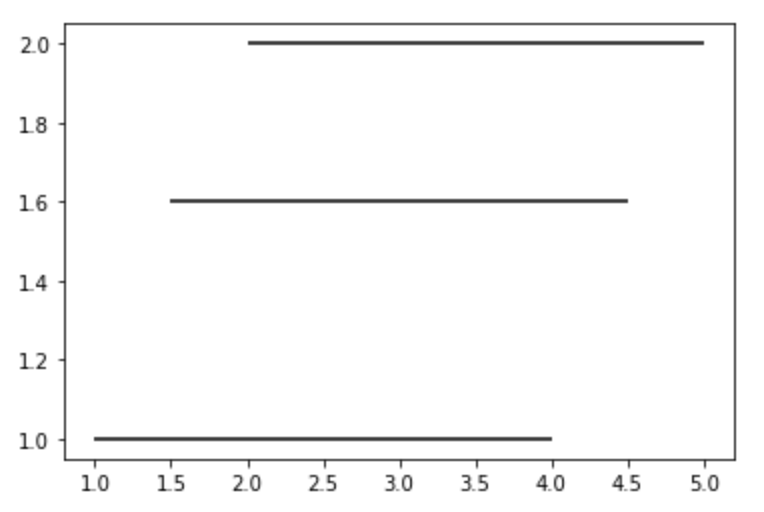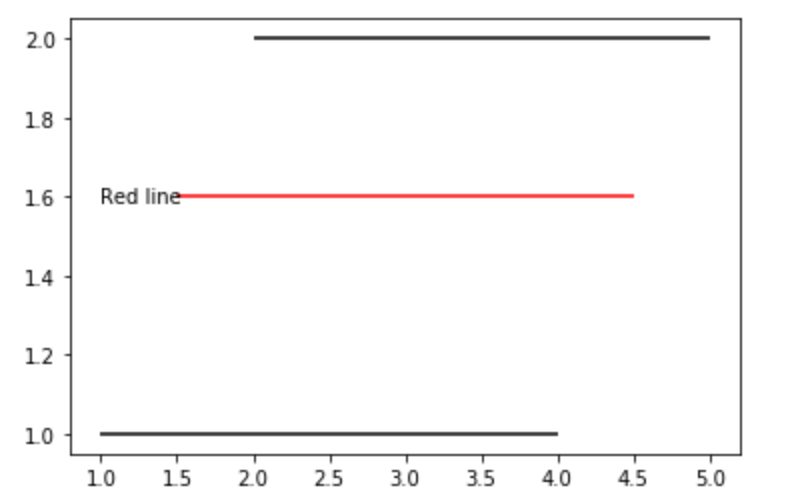GeeksforGeeks App
Open AppBrowser
Continue

# Matplotlib.pyplot.hlines() in Python

Matplotlib is an amazing visualization library in Python for 2D plots of arrays. Matplotlib is a multi-platform data visualization library built on NumPy arrays and designed to work with the broader SciPy stack.

## Matplotlib.pyplot.hlines()

The `Matplotlib.pyplot.hlines()` is used to draw horizontal lines in a graph at each y from xmin to xmax.

Syntax: matplotlib.pyplot.hlines(y, xmin, xmax, colors=’k’, linestyles=’solid’, label=”, *, data=None, **kwargs

Parameters:
The `Matplotlib.pyplot.hlines()` accepts the below-described parameters:

• y : It is a required parameter for this method. This parameter describes that in the graph the line is to be drawn. Its value is a scalar or sequence of scalars, in other words, it is the y-indexes where the line is to be plotted.
• xmin: It is a required parameter that has either a scalar value or a 1D array-like value that sets the beginning of each line. If scalars are provided all the lines will have the same length.
• colors: As the name suggests it is used to set the color of the line to be plotted. This parameter is optional in nature and its default value is ‘k’
• linestyles: It is also an optional parameter that accepts four values namely ‘solid’, ‘dashed’, ‘dashdot’ and ‘dotted’. It is responsible for setting the style of the line to be plotted.
• label: It is an optional parameter used to describe information about the plotted line in the same line. This accepts a string whose default value is an empty string.
• **kwargs: This parameter is used to make use of LineCollection properties in the plotted line.

Note: In addition to the above-mentioned parameters, this method can take a data keyword argument. It is also important to note that the object passed as data must support item access and membership test.

Example 1:

 `from` `matplotlib ``import` `pyplot as plt`` ` `plt.hlines(y ``=` `1``, xmin ``=` `1``, xmax ``=` `4``)`` ` `plt.hlines(y ``=` `1.6``, xmin ``=` `1.5``, xmax ``=` `4.5``)`` ` `plt.hlines(y ``=` `2``, xmin ``=` `2``, xmax ``=` `5``)`

Output :Example 2:

 `from` `matplotlib ``import` `pyplot as plt`` ` `plt.hlines(y ``=` `1``, xmin ``=` `1``, xmax ``=` `4``, label ``=``"black line"``)`` ` `plt.hlines(y ``=` `1.6``, xmin ``=` `1.5``, xmax ``=` `4.5``, color ``=``'r'``)``plt.text(``1``, ``1.6``, ``'Red line'``, ha ``=``'left'``, va ``=``'center'``)`` ` `plt.hlines(y ``=` `2``, xmin ``=` `2``, xmax ``=` `5``)`

Output :My Personal Notes arrow_drop_up